# CW implies paracompact Hausdorff

This article gives the statement and possibly, proof, of an implication relation between two topological space properties. That is, it states that every topological space satisfying the first topological space property must also satisfy the second topological space property
View all topological space property implications | View all topological space property non-implications
|
Property "Page" (as page type) with input value "{{{stronger}}}" contains invalid characters or is incomplete and therefore can cause unexpected results during a query or annotation process.
Property "Page" (as page type) with input value "{{{weaker}}}" contains invalid characters or is incomplete and therefore can cause unexpected results during a query or annotation process.

This article involves a proof using cellular induction, viz, it inductive construction on the$n$-skeleton of a cellular space

## Statement

Every CW-space (viz., every space which can be given a CW-complex structure) is a paracompact Hausdorff space.

## Proof

### Goal of the proof

Let$X$ be a CW-space. Equip$X$ with a CW-complex structure and let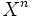$X^n$ denote the$n$-skeleton with respect to that structure.

Let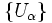$\left \{ U_\alpha \right \}$ be an open cover for$X$.

The goal is to constrict a partition of unity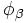$\phi_\beta$ on$X$, subordinate to the open cover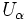$U_\alpha$.

We shall do this inductively on the$n$-skeleton. In other words, we shall construct a partition of unity on$X^n$, and extend it to a partition of unity on$X^{n+1}$.

### Proof details

Suppose we have constructed$\phi_\beta$ on the$n$-skeleton. The goal is to now extend it to the$n+1$-skeleton.

It suffices to describe how to extend$\phi_\beta$ for one specific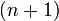$(n+1)$-cell. The question boils down to the following:

Given a partition of unity on the boundary of a disc, which is subordinate to the intersection of an open cover with the boundary, extend it to a partition of unity on the whole disc, which is subordinate to the open cover on the whole disc.

The idea in performing this extension is to observe that any open set of the boundary has some thickness about the boundary (using compactness of the boundary) and any function whose support is inside the open set can be extended so that the support of the function on the whole disc, still lies inside the open set.